Courses

# Revisal Problems (Past 13 Years) JEE Main (Chemical Bonding)

## 24 Questions MCQ Test Chemistry for JEE Advanced | Revisal Problems (Past 13 Years) JEE Main (Chemical Bonding)

Description
This mock test of Revisal Problems (Past 13 Years) JEE Main (Chemical Bonding) for JEE helps you for every JEE entrance exam. This contains 24 Multiple Choice Questions for JEE Revisal Problems (Past 13 Years) JEE Main (Chemical Bonding) (mcq) to study with solutions a complete question bank. The solved questions answers in this Revisal Problems (Past 13 Years) JEE Main (Chemical Bonding) quiz give you a good mix of easy questions and tough questions. JEE students definitely take this Revisal Problems (Past 13 Years) JEE Main (Chemical Bonding) exercise for a better result in the exam. You can find other Revisal Problems (Past 13 Years) JEE Main (Chemical Bonding) extra questions, long questions & short questions for JEE on EduRev as well by searching above.
QUESTION: 1

### Direction (Q. Nos. 1-20) This section contains 20 multiple choice questions. Each question has four choices (a), (b), (c), and (d), out of which ONLY ONE option is correct Q. Some of the following compounds have more than one type of bonding. KCN, NH4CI, NaCI, H2O, H2SO4,CaO, MgCI2, CH4, H2 These are

Solution: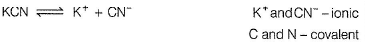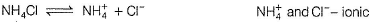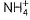has three covalent and one coordinate bond.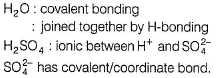QUESTION: 2

### Out of the following paramagnetic species are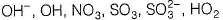Solution:

Species with odd number of electrons will certainly have unpaired electron in bonding, thus paramagnetic.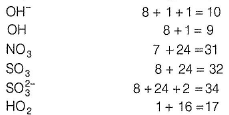Thus, OH, NO3 and HO2 are paramagnetic.

QUESTION: 3

### The Cl— C— Cl angles in I. 1,1,2, 2-dichloroethene II. tetrachloromethane and III. 1, 1-dichloroelhane respectively will be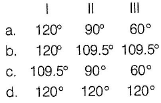Solution: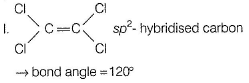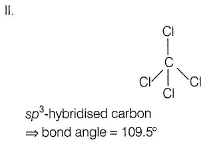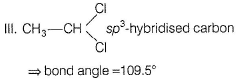QUESTION: 4

Metallic bonding is explained by

Solution:

Bonding in metals is called metallic bonding. It results from the electrical attractions among positively charged metal ions and mobile, delocalised electrons belonging to the crystal as a whole. Two theories:
(a) Band metal
(b) Electron-sea model

QUESTION: 5

The shape of IF7 molecule is

Solution:

l-atom is sp3d3 -hybridised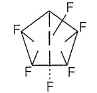Bond angle = 72° {for five) and = 90° (for two)
No lone pair

QUESTION: 6

AsF5 molecule is trigonal bipyramidal. The hybrid orbitals used by As atoms for bonding are

Solution: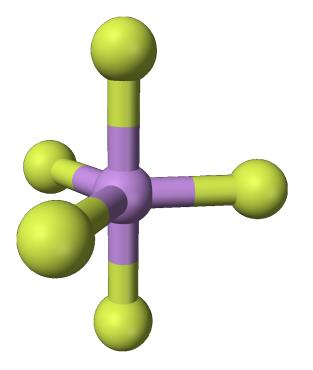This is the structure for AsF5 which is Trigonal Bipyramidal, it has sp3d hybridization.

So, s and all three orbitals of p will be used. Since the bonds with the remaining two Fs are in the perpendicular plane, when we compare it to the shape of different d- orbitals, we get dz2 as the answer.

Hence C is the correct answer.

QUESTION: 7

P4 is stable form of phosphorus.The percentage of p - character in the orbital forming (P—P) bonds in P4 is

Solution: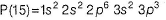Valence electrons = 5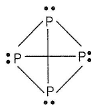Each P-atom has three (P— P) bonds and one lone pair and is sp3-hybridised.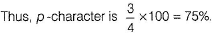QUESTION: 8

Central O-atom in O3 is ...... hybridised.

Solution: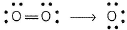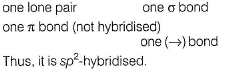QUESTION: 9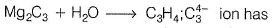Solution: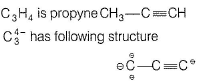Thus, two σ and two π-bonds.

QUESTION: 10

Among the following species,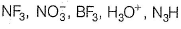isostructural pairs are

Solution: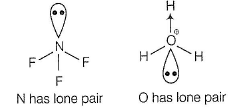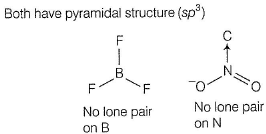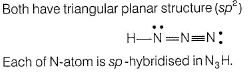QUESTION: 11

If there are five electron pairs in outer shell of an atom, then structure and bond angle as predicted by Sidgwick-Powell theory is

Solution:

There are five bonding pairs or electron pairs. Thus, sp3d-hybridisation. Trigonal bipyramidal structure.
Three bonding pairs are at 120° with one each other.
Two bonding pairs are at 90° with the plane in which first three bonding pairs exist.

QUESTION: 12

Consider the following molecules

I. Acetic acid
II. o-nitrophenol
III. m-nitrophenol
IV. o-boric acid

Select the species showing intermolecular (X)and intermolecular the H-bonding (Y).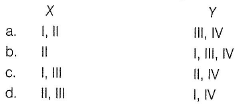Solution: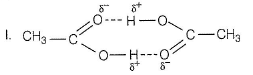Interamolecular H-bonding (dimer is formed).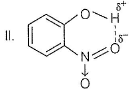Intramolecular H-bonding (makes it more volatile than m-and p-isomers)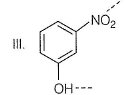Intermolecular H-bonding.
IV. H3BO3 : Hexagonal structure is formed due to intermolecular H-bonding.

QUESTION: 13

In which of the following molecules would you expect the nitrogen-to-nitrogen bond to be the shortest?

Solution: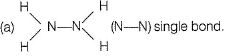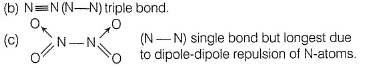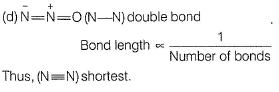QUESTION: 14

Chelation can occur in

Solution:

(a) Chelation is ring formation.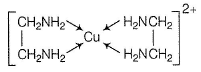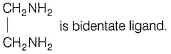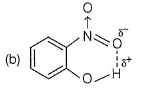Intramolecular H-bonding.

QUESTION: 15

The correct order of dipole moment of HF, H2S and H2O molecules is in order.

Solution: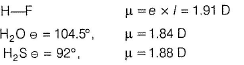Thus, HF > H2S > H2O

QUESTION: 16

Solubility of the salts of group IIA (alkaline earth metals) is in orde

Solution:

Going down the group, size of the cation increases. Since, the lattice energy decreases much more than the hydration energy with increasing size solubility of hydroxide increases (when size of cation and anion are of comparable size). (b) and (c) If the anion is large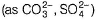com p are d to cation, the lattice energy will remain almost constant. Since, hydration energy decreases down a group, solubility will decrease hence (b) and (c) are incorrect.

QUESTION: 17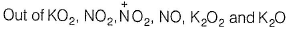unpaired ele ctrons are present in

Solution:

KO2 has superoxide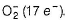Thus, one electron is upaired.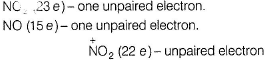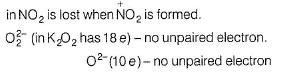QUESTION: 18

EN values of elements are given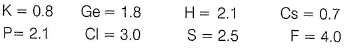Q. Out of KCI, PH3, GeCI, H2S and CsF ionic compounds are

Solution:

Compound is said to be ionic if (EN) difference between bonding atoms exceeds 1.7.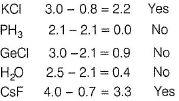(Note CsF is most ionic.}

QUESTION: 19

Ionisation potential of sodium is (5.1 eV) and the electron affinity of chlorine is (3.6 eV). Working Space Energy required to transfer one electron from and an isolated sodium atom to an isolated gaseous atom is

Solution: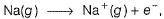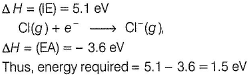QUESTION: 20

Consider the following complexes.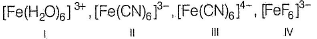Q. Fe is sp3d2 hybridisation in w hich of the above complexes

Solution:

H2O and F- are weak ligands. Hence, unpaired electrons in Fe3+ is (I) and (IV) are not affected. Thus outer d-orbitals are involved in hybridisation.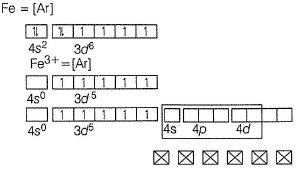Six sp3d2-orbitals accept lone pair from weak ligands - octahedral geometry.

QUESTION: 21

Direction (Q. Nos. 21-24) This section is based on statement I and statement II. Select the correct answer from the codes given below.

Q.

Statement I : The chlorine to oxygen bond distance in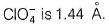Statement II : There must be considerable double bond character.

Solution: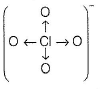There are (Cl → O) coordinate bonds. Thus, bond length is shorter than the normal (Cl—O) bond length (1.72A°). Thus, there is considerable double bond character. Thus, both statements I and II are correct and statement II is the correct explanation statement I.

QUESTION: 22

Statement I : (N—N) bond length in N2O4 is larger than normal (N—N) bond length in N2H4.

Statement I : I Nitrogen completes its octet in N2O4.

Solution:

N2O4 is formed by dimerisation of NO2.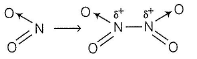Unpaired electron is shared and (N—N) bond is formed. This results is formation of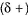on each N-atom. Thus, dipole-dipole repulsion increases (N—N) bond length (larger than its normal value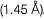. Thus, both statements I and II are correct but statement II is not the correct explanation of statement I.

QUESTION: 23

Statement I :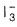is linear in structure.

Statement II : l2 is a Lewis acid and l- is a Lewis base.

Solution: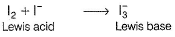There are two bond pairs and three lone pairs in the outer shell of central atom. To minimise the repulsive forces, the three lone pairs occupy the equatorial position. The ion is therefore linear in shape with a bond angle of exactly 180°.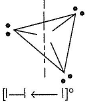Thus, both statements I and II are correct but statement II is not the correct explanation of statement I,

QUESTION: 24

Statement I :is form ed but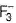is not formed.

Statement II : F- is not a Lewis base.

Solution:andare of same group. I can expand its octet but F cannot expand its octet, thus inoctet rule is not violated but in, octet rule is violated.
F- (electron rich) is a Lewis base.
Thus, statement I is correct and statement II is incorrect.